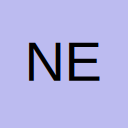# Add Formula to Cell With VBA Macro Code

• Hello there. Can anyone please tell me what's wrong with the following vba code?

Code
``````Range("B16").Formula = "=DATE(YEAR(" & Cells(16,1) & ",MONTH(" & Cells(16,1) +1 & ",0)""

where Cells(16,1) = "Feb-2007"``````

Cheers.

• Re: Create A Formula To A Cell By Vba

think you're missing a bracket....try

Code
``Range("B16").Formula = "=DATE(YEAR(" & Cells(16,1) & "),MONTH(" & Cells(16,1) +1 & "),0)"``

D:sailboat: [SIZE=1]Better a bad day on the water than a good day in the office[/SIZE]

• Re: Add Formula to Cell With VBA Macro Code

Hi there. I tried the following modified code:

Code
``````Range("B16").Formula = "=DATE(YEAR(" & Cells(16, 1) & "),MONTH(" & Cells(16, 1) + 1 & "),0)"
'where cells(16,1).value="Feb-2007"``````

but the formula in the cell turned out to be:
=DATE(YEAR(1/2/2007),MONTH(2/2/2007),0)
and the result : 00/01/1900

• Re: Add Formula to Cell With VBA Macro Code

Not sure what you mean - the formula does what you'd asked for...

however...if you are trying to get a reference to the cell in the formula...try

Code
``Range("B16").Formula = "=DATE(YEAR(R16C1),MONTH(R16C1),0)"``

If you are using R1C1 cell reference or

Code
``Range("B16").Formula = "=DATE(YEAR(\$A\$16),MONTH(\$A\$16),0)"``

if not

D:sailboat: [SIZE=1]Better a bad day on the water than a good day in the office[/SIZE]

• Re: Add Formula to Cell With VBA Macro Code

Sorry. I've got a little muddle myself! The required formula should be created in range("B16) and the cell(r,c) should be the cell "A16" where Feb-2007 was stored.

Sorry for that!

## Participate now!

Don’t have an account yet? Register yourself now and be a part of our community!